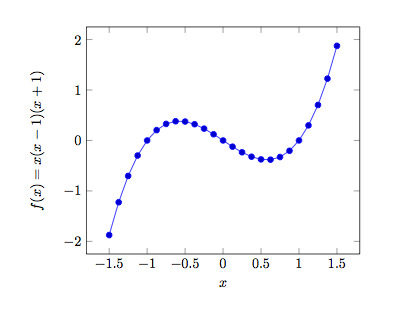# Newton Raphson Works Great, Until It Doesn't - Part 1

Calculus Level 4Consider the function given by $f(x) = x(x-1)(x+1)$. If we apply the Newton Raphson method with the approximate root $x_0 = 0.45$, which root would we converge to?

×